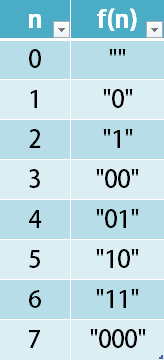##### Welcome to Subscribe On Youtube

Formatted question description: https://leetcode.ca/all/1256.html

# 1256. Encode Number

Medium

## Description

Given a non-negative integer num, Return its encoding string.

The encoding is done by converting the integer to a string using a secret function that you should deduce from the following table:Example 1:

Input: num = 23

Output: “1000”

Example 2:

Input: num = 107

Output: “101100”

Constraints:

• 0 <= num <= 10^9

## Solution

If n is 0, then f(n) is "". If 1 <= n < 3, then f(n) is a binary string with length 1. If 3 <= n < 7, then f(n) is a binary string with length 2. If 7 <= n < 15, then f(n) is a binary string with length 3.

The encoding function is as follows. For the given number num, obtain the number of bits bits for number num + 1, where the number of bits means the number of bits remaining after removing leading zeros, calculate difference as the difference between num + 1 and 2 ^ bits, and return the binary representation of difference.

• class Solution {
public String encode(int num) {
num++;
int bits = (int) (Math.log(num) / Math.log(2));
int difference = num - (int) Math.pow(2, bits);
String encodeStr = binary(difference, bits);
return encodeStr;
}

public String binary(int num, int bits) {
String str = "";
for (int i = 0; i < bits; i++) {
int remainder = num % 2;
str = remainder + str;
num /= 2;
}
return str;
}
}

• // OJ: https://leetcode.com/problems/encode-number/
// Time: O(logN)
// Space: O(logN)
class Solution {
public:
string encode(int n) {
++n;
string ans;
int len = log(n);
while (n) {
ans += '0' + (n & 1);
n >>= 1;
}
if (ans.size() > len) ans.pop_back();
return string(rbegin(ans), rend(ans));
}
};

• class Solution:
def encode(self, num: int) -> str:
return bin(num + 1)[3:]


• func encode(num int) string {
num++
s := strconv.FormatInt(int64(num), 2)
return s[1:]
}

• function encode(num: number): string {
++num;
let s = num.toString(2);
return s.slice(1);
}

`